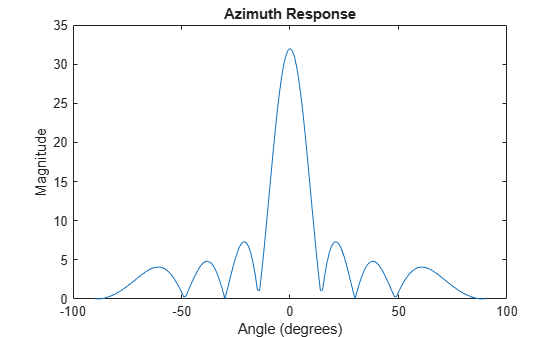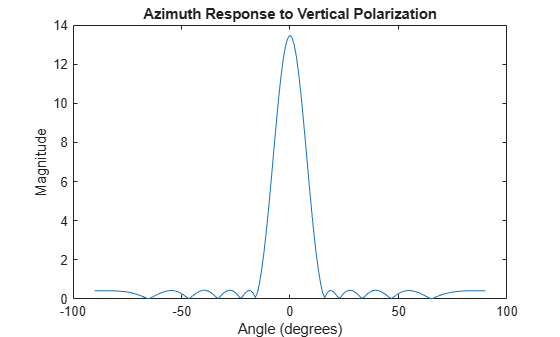# phased.SphericalWavefrontArrayResponse

Array response to spherical wavefront

## Description

The `phased.SphericalWavefrontArrayResponse` System object™ calculates the complex-valued response of a sensor array to a spherical wave.

To compute the response:

1. Create the `phased.SphericalWavefrontArrayResponse` object and set its properties.

2. Call the object with arguments, as if it were a function.

## Creation

### Syntax

``response = phased.SphericalWaveformArrayResponse``
``response = phased.SphericalWaveformArrayResponse(Name,Value)``

### Description

example

```The `response = phased.SphericalWaveformArrayResponse` creates a `phased.SphericalWaveformArrayResponse` System object `response` with default property values.```
````response = phased.SphericalWaveformArrayResponse(Name,Value)` creates a `phased.SphericalWaveformArrayResponse` object with each property `Name` set to a specified `Value`. You can specify additional name-value pair arguments in any order as (`Name1`,`Value1`,...,`NameN`,`ValueN`). Enclose each property name in single quotes.Example: ```response = phased.SphericalWaveformArrayResponse('SensorArray',phased.URA([10,20],'ElementSpacing',[0.25,0.25]),'PropagationSpeed',physconst('LightSpeed'))``` creates a `phased.SphericalWaveformArrayResponse` System object for a 10-by-20 uniform rectangular array (URA) with element spacing set to 0.25 meters The propagation speed set to the speed of light.```

## Properties

expand all

Unless otherwise indicated, properties are nontunable, which means you cannot change their values after calling the object. Objects lock when you call them, and the `release` function unlocks them.

If a property is tunable, you can change its value at any time.

Sensor array, specified as a Phased Array System Toolbox array System object.

Example: `phased.URA`

Signal propagation speed, specified as a positive scalar. Units are in meters per second. The default propagation speed is the value returned by `physconst('LightSpeed')`. See `physconst` for more information.

Example: `3e8`

Data Types: `double`

Option to include individual element responses in the steering vector, specified as `false` or `true`. If this property is set to `true`, the steering vector includes individual array element responses. If this property is set to `false`, the steering vector is computed assuming that the elements are isotropic, regardless of how the elements are specified. Set this property to true when using polarized signals.

When the array specified in the SensorArray property contains subarrays, the steering vector applies to subarrays. If SensorArray does not contain subarrays, the steering vector applies to the array elements.

Data Types: `logical`

Enable weights input, specified as `false` or `true`. When `true`, use the object input argument `W` to specify weights. Weights are applied to individual array elements (or at the subarray level when subarrays are supported).

Data Types: `logical`

Enable polarization simulation, specified as `false` or `true`. Set this property to `true` to enable polarization and to `false` to ignore polarization. This property applies when the array specified in the `SensorArray` property is capable of simulating polarization and the `IncludeElementResponse` property of the array is set to `true`.

Data Types: `logical`

## Usage

### Syntax

``RESP = response(FREQ,ANG,RNG)``
``RESP = response(FREQ,ANG,RNG,W)``

### Description

example

````RESP = response(FREQ,ANG,RNG)` returns the array response `RESP` at operating frequencies specified in `FREQ`, directions specified in `ANG`, and at range `RNG`.```

example

````RESP = response(FREQ,ANG,RNG,W)` applies weights `W` to the sensor array. To enable this syntax, set the `WeightsInputPort` to `true`.```

### Input Arguments

expand all

Frequencies for computing directivity and patterns, specified as a positive scalar or 1-by-L real-valued row vector. Frequency units are in hertz.

• For an antenna, microphone, or sonar hydrophone or projector element, `FREQ` must lie within the range of values specified by the `FrequencyRange` or `FrequencyVector` property of the element. Otherwise, the element produces no response and the directivity is returned as `–Inf`. Most elements use the `FrequencyRange` property except for `phased.CustomAntennaElement` and `phased.CustomMicrophoneElement`, which use the `FrequencyVector` property.

• For an array of elements, `FREQ` must lie within the frequency range of the elements that make up the array. Otherwise, the array produces no response and the directivity is returned as `–Inf`.

Example: `[1e8 2e6]`

Data Types: `double`

Angles for computing response, specified as a 1-by-M real-valued row vector or a 2-by-M real-valued matrix, where M is the number of angular directions. Angle units are in degrees. If `ANGLE` is a 2-by-M matrix, then each column specifies a direction in azimuth and elevation, `[az;el]`. The azimuth angle must lie between –180° and 180°. The elevation angle must lie between –90° and 90°.

If `ANGLE` is a 1-by-M vector, then each entry represents an azimuth angle, with the elevation angle assumed to be zero.

The azimuth angle is the angle between the x-axis and the projection of the direction vector onto the xy plane. This angle is positive when measured from the x-axis toward the y-axis. The elevation angle is the angle between the direction vector and xy plane. This angle is positive when measured towards the z-axis. See Azimuth and Elevation Angles.

Example: `[45 60; 0 10]`

Data Types: `double`

Range, specified as a positive 1-by-M real-valued vector of positive values. If `ANG` has more than one column, `RNG` must be a scalar or have the same number of columns as `ANG`. Units are in meters.

Data Types: `double`

Weights applied to sensor array elements, specified as a complex-valued, length-N column vector or a complex-valued, N-by-L matrix. N is the number of array elements and L is the number of frequencies specified in `FREQ`. If `W` is a vector, the same weight is applied at all frequencies. If `W` is a matrix, each column of `W` represents the weights used at the corresponding frequency specified in `FREQ`.

#### Dependencies

To enable this argument, set the `WeightsInputPort` to `true`.

Data Types: `double`
Complex Number Support: Yes

### Output Arguments

expand all

Array response, returned as a complex-valued M-by-L complex-valued matrix or `struct` containing complex values. The response depends on whether the `EnablePolarization` property is set to `true` or `false`.

• If the `EnablePolarization` property is set to `false`, the voltage response, `RESP`, has the dimensions M-by-L. M represents the number of angles specified in the input argument `ANG` while L represents the number of frequencies specified in `FREQ`.

• If the `EnablePolarization` property is set to `true`, the voltage response, `RESP`, is a MATLAB® `struct` containing two complex fields, `RESP.H` and `RESP.V`. The `RESP.H` field represents the array’s horizontal polarization response, while `RESP.V` represents the array’s vertical polarization response. Each field has the dimensions M-by-L. M represents the number of angles specified in the input argument, `ANG`, while L represents the number of frequencies specified in `FREQ`.

Data Types: `double`
Complex Number Support: Yes

## Object Functions

To use an object function, specify the System object as the first input argument. For example, to release system resources of a System object named `obj`, use this syntax:

`release(obj)`

expand all

 `step` Run System object algorithm `release` Release resources and allow changes to System object property values and input characteristics `reset` Reset internal states of System object

## Examples

collapse all

Calculate and plot the azimuth response of a 4-by-8 uniform rectangular array at directions between $-9{0}^{\circ }$ and $9{0}^{\circ }$ in azimuth at a range of 1 km. Assume the array operating frequency is 300 MHz.

Create the array and the `phased.SphericalWavefrontArrayResponse System object™`.

``` array = phased.URA([4,8]); response = phased.SphericalWavefrontArrayResponse( ... 'SensorArray',array); ang = -90:90; rng = 1000.0; fc = 300e6;```

Compute and plot the response as a function of azimuth angle.

``` resp = response(fc,ang,rng); plot(ang,abs(resp)) xlabel('Angle (degrees)') ylabel('Magnitude') title('Azimuth Response')```Calculate and plot the azimuth response of an 11-element uniform linear array at directions between $-9{0}^{\circ }$ and $9{0}^{\circ }$ in azimuth at a range of 1 km. Create a polarized field using short-dipole antenna elements. Assume the array operating frequency is 300 MHz.

Create the ULA array and the `phased.SphericalWavefrontArrayResponse System object™.`

```antenna = phased.ShortDipoleAntennaElement; array = phased.ULA(11,'Element',antenna); response = phased.SphericalWavefrontArrayResponse( ... 'SensorArray',array,'EnablePolarization', ... true,'WeightsInputPort',true); ang = -90:90; rng = 1000.0; fc = 300e6; w = taylorwin(11);```

Compute and plot the response as a function of azimuth angle.

```resp = response(fc,ang,rng,w); plot(ang,abs(resp.V)) xlabel('Angle (degrees)') ylabel('Magnitude') title('Azimuth Response to Vertical Polarization')```## Version History

Introduced in R2021b